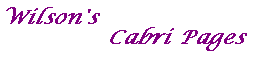#### Proof of Theorem 2

Theorem 2
Suppose that C: xTMx=0 is a non-degenerate conic and that VW is a chord of C passing through a fixed point U.
Then the tangents at V and W meet on the algebraic polar of U with respect to C.

Proof
By Theorem 1, the tangents at V=[v] and W=[w] have equations vTMx=0 and wTMx=0, respectively.
Suppose that these meet in T=[t]. By definition, T is on the geometrical polar of U.
As VW passes through U=[u], u = av + bw, for some a, b.
Then uTMt=avTMt+wTMt=0 since T lies on each tangent.
Thus T lies on uTMx=0,the algebraic polar of U.